# Spiroshapes aka Drawing Curved Shapes (2007/03/14)

## Recommended Posts

SpiroShapes aka Drawing Curved ShapesWhat's this?

Based directly on BoltBait code for his Polygon.dll,

another way to draw amazing lines/curves:

EDIT: Old CodeLab code replaced by plugin presentation!

I've called it SpiroShapes, because it makes me think about the 'drawing machine' Spirograf.

Some examples...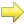here is the DLL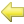The MadJik's All plugins package is available !

How to install

Close Paint.net

Classic version of Paint.net

Unzip and (re)place the DLL in your Effect folder usually: C:/Program Files/Paint.NET/Effects

Microsoft Store version of Paint.net

Unzip and (re)place the DLL in your Effect folder usually: /My Documents/paint.net App Files/Effects/

The User interface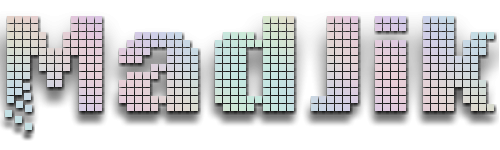My DeviantArt | My Pictorium | My Plugins | Donate via Paypal

##### Share on other sites

Madjik, while clearly fascinating, how do you predict what will happen when you adjust the various inputs?

In other words, in the original final script, you can clearly guess that when you change verticies from 5 to 6, you can guess that you will go from drawing a pentagon to a hexagon. In yours, increasing verticies makes the curves smoother.

But, what is coefficient a, b, and c? What do they 'mean'?

Getting the specified line width from the UI is easy:

`int LineWidth = (int)EnvironmentParameters.BrushWidth;`

As for how to modify my line drawing routine to take advantage of it (AND antialias the resulting line), now that's another story.

##### Share on other sites

Well, I'm not able to explain sin/cos here in english...

Also it is difficult to pre-evaluate the curve we will obtain when we change CoefA or CoefB or CoefC.

First formula is : RO = sin( CoefA * ANGLE / CoefB)

This is a simple sinus curve.

Then to draw a circle we need to calculate X,Y

Radius is normaly a "fix" value.

if you put that in a loop for ANGLE = -PI to PI you could plot a circle.

It means the radius is changing at each plot...

CoefA is the number of period for the sinus curve

(in a period sin goes like this : 0...-1...0...1...0). Each -1 or 1 is a peak.

If CoefA = 2 then we have 4 peaks. 6 : 12 peaks, etc...

CoefB is a decal factor. CoefB=1 : no decal. You could see that I'm calling the peaks !

Experiment differentes values to feel how it works...

Test (CoefC = CoefB):

CoefA=2 CoefB=1

CoefA=6 CoefB=1

CoefA=7 CoefB=6

Change the type from Integer to Float and test

CoefA=2 CoefB=0.5

CoefA=1.5 CoefB=1 (the fly)

CoefC should be equal to CoefB if we want to have all periods finished.

If we choose CoefC < CoefB then we obtain an "open" curve.

If we choose CoefC > CoefB then we redraw on the same plots. It's too much plots.

NB: In the formula we have : CoefA / CoefB

It means CoefA=1/CoefB=2 is same as CoefA=2/CoefB=4 ,CoefA=3/CoefB=6, etc...

I have an excel file with the graph function PM me if you want it...

I have used some curves and polar inversion to do this:

http://paintdotnet.12.forumer.com/viewtopic.php?p=16898#16898My DeviantArt | My Pictorium | My Plugins | Donate via Paypal

##### Share on other sites

Well, I make my first GUI using Visual Studio 2005 Express (free).

I took other source (Clouds Effect) as model because C# isn't a language I know.

Now, it seems to work but I have an error if the image is too small (index out of bound)... and the preview does show the full lines (final rendering also... but last display is Ok).

I would appreciate if someone could have a look at the source and fix the bugs for me. After that I will create explanation for this plug-in....

here is the DLL

here is the sourceMy DeviantArt | My Pictorium | My Plugins | Donate via Paypal

##### Share on other sites

I crashed Paint.NET just now using the Theta curves plugin. I think this is the error you were talking about. It happened when I set the vertices's to "0".

Here is the crash log:

This text file was created because Paint.NET crashed.

Please e-mail this file to paint.net@hotmail.com so we can diagnose and fix the problem.

Application version: Paint.NET v3.0 (Alpha 3 Release build 3.0.2500.35765)

Time of crash: 11/22/2006 9:25:41 AM

Application uptime: 00:00:23.9064030

OS Version: 5.1.2600.131072 Service Pack 2 Workstation x86

.NET Framework version: 2.0.50727.42 x86

Processor: 1x Intel® Pentium® 4 CPU 2.66GHz

Physical memory: 511 MB

Locale: pdnr.c: en-US, hklm: en-US, hkcu: n/a, cc: en-US, cuic: en-US

Exception details:

System.ArgumentOutOfRangeException: Value of '1' is not valid for 'Value'. 'Value' should be between 'Minimum' and 'Maximum'.

Parameter name: Value

at System.Windows.Forms.NumericUpDown.set_Value(Decimal value)

at PaintDotNet.Effects.ThetaCurvesConfigDialog.trackbar_Vertices_ValueChanged(Object sender, EventArgs e)

at System.Windows.Forms.TrackBar.OnScroll(EventArgs e)

at System.Windows.Forms.TrackBar.WndProc(Message& m)

at System.Windows.Forms.Control.ControlNativeWindow.OnMessage(Message& m)

at System.Windows.Forms.Control.ControlNativeWindow.WndProc(Message& m)

at System.Windows.Forms.NativeWindow.Callback(IntPtr hWnd, Int32 msg, IntPtr wparam, IntPtr lparam)

------------------------------------------------------------------------------

Take responsibility for your own intelligence.-Rick Brewster

##### Share on other sites

There is a reason for the following comment in the original code:

```    int verticies = 5;        // number of points (3-10?), default 5, slider
```

The range for verticies can not go below 3. Actually, it can not go below 1. If Verticies is 0 you will get a division by zero error.

##### Share on other sites
There is a reason for the following comment in the original code:

```    int verticies = 5;        // number of points (3-10?), default 5, slider
```

The range for verticies can not go below 3. Actually, it can not go below 1. If Verticies is 0 you will get a division by zero error.

For the CodeLab on this topic, you are right !

And of course if vertice is 0 we get a division by zero error.

But with vertice 1 or 2 the code is useless cos we get a single point or a line...My DeviantArt | My Pictorium | My Plugins | Donate via Paypal

##### Share on other sites
Well, I make my first GUI using Visual Studio 2005 Express (free).

I took other source (Clouds Effect) as model because C# isn't a language I know.

Now, it seems to work but I have an error if the image is too small (index out of bound)... and the preview does show the full lines (final rendering also... but last display is Ok).

I would appreciate if someone could have a look at the source and fix the bugs for me. After that I will create explanation for this plug-in....

here is the DLL

here is the source

Updated !

I changed "Double" to "Float" and it is a bit faster now. Some bugs corrected as well.

I still need your help for the bug I get when it is small size image...

I test with image size 600x600 px and it is Ok... This is an example after some works...

I still like spheres...

I used my ThetaCurves DLL (beta version with some bugs)...

http://paintdotnet.12.forumer.com/viewtopic.php?p=17314#17314

Also the gradient effect and the new distorb sphere effect...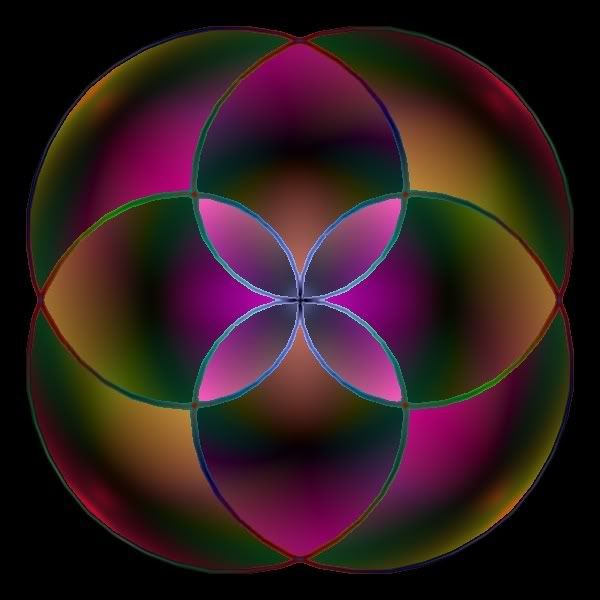(ok need some more blur...)My DeviantArt | My Pictorium | My Plugins | Donate via Paypal

##### Share on other sites

:wink: Thanks ! Madjik ! Nice work !

To be free To be wild

And to be Just like a child

Mike Oldfield

http://www.myspace.com/tubulartos

##### Share on other sites
• 2 weeks later...This picture has been made using the Polygones.dll

here is the Polygones.DLL

__________________________

Also, I have made some changes to fix bugs on the ThetaCurves.DLL

here is the DLL

here is the source

Bugs fixed:

• Missing dot in some curves making the fill effect useless...
Tiling is now working. Advice is to use the Dot mode (Line mode takes too long time)
Since Paint.net V3.0 the error for small pictures seams self corrected!

Known Bugs:

• Preview isn't shown perfectly...

==> to BoltBait :

Could you change the topic title (Codelab + Plugin...)?My DeviantArt | My Pictorium | My Plugins | Donate via Paypal

##### Share on other sites
• 5 weeks later...

MadJik: you should add the anti-aliasing function of your Oblique plugin to the Theta curves and Polygons plugins...

Take responsibility for your own intelligence.-Rick Brewster

##### Share on other sites

It is (quite) easy to use the standard pre-programmed GUI for 1,2 or 3 sliders...

When we need something else than sliders or more than 3 the challenge is more difficult (for me).

I'll miss time next days for that. So it will be done in 1-2 weeks...My DeviantArt | My Pictorium | My Plugins | Donate via Paypal

##### Share on other sites
• 2 weeks later...I have made some changes on the ThetaCurves.DLL

It's faster now!

here is the DLL

///////////////

I can't find a way to add anti-alias on Polygone and Thetacurve effects... So if someone could be kind to point me some code for that...

///////////////

Also I can't find the code to organize the submenu plugins (it seems simple for Rick, but for me I need a source code)... So if someone could be kind to point me some code for that too...

EDIT: I've find a solution in the paint.net source v2.72 : Text search on the full folder "submenu" point me out the bulge code with the solution in!My DeviantArt | My Pictorium | My Plugins | Donate via Paypal

##### Share on other sitesFaster !

here is the DLL

____________________________________________it's now calles Spiro-Shapes Effect

Faster !

here is the DLL

____________________________________________

To install:

Close Paint.net

Unzip and (re)place the DLL in your Effect folder usualy: C:\Program Files\Paint.NET\Effects

You could delete the Thetacurves.dll if it exists!My DeviantArt | My Pictorium | My Plugins | Donate via Paypal

##### Share on other sites

Have you added anti-aliasing to the effects?

Take responsibility for your own intelligence.-Rick Brewster

##### Share on other sites
Have you added anti-aliasing to the effects?

No, sorry! The methode I thought about isn't working well for that!

I would appreciate if someone could help for this part!My DeviantArt | My Pictorium | My Plugins | Donate via Paypal

##### Share on other sites

A conceptional star:vb code illustrates

```Imports System.Drawing
Imports System.Drawing.Drawing2D
Imports System.Drawing.Imaging
Imports System.Text

'option strict off

Public Class Form1
Inherits System.Windows.Forms.Form

#Region " Windows Form Designer generated code "

Public Sub New()
MyBase.New()

'This call is required by the Windows Form Designer.
InitializeComponent()

'Add any initialization after the InitializeComponent() call

End Sub

'Form overrides dispose to clean up the component list.
Protected Overloads Overrides Sub Dispose(ByVal disposing As Boolean)
If disposing Then
If Not (components Is Nothing) Then
components.Dispose()
End If
End If
MyBase.Dispose(disposing)
End Sub

'Required by the Windows Form Designer
Private components As System.ComponentModel.IContainer

'NOTE: The following procedure is required by the Windows Form Designer
'It can be modified using the Windows Form Designer.
'Do not modify it using the code editor.
Private Sub InitializeComponent()
'
'Form1
'
Me.AutoScaleBaseSize = New System.Drawing.Size(5, 13)
Me.ClientSize = New System.Drawing.Size(592, 573)
Me.Name = "Form1"
Me.Text = "Form1"

End Sub

#End Region
Private Sub form1_paint(ByVal sender As Object, ByVal e As PaintEventArgs) Handles MyBase.Paint
Dim gr As Graphics = e.Graphics

gr.Clear(Color.Azure)
Dim n As Integer = 5
Dim a As Double = 0.0
Dim b As Double = 0.0
Dim R1 As Integer = 250 'CInt(Math.Min(Me.ClientSize.Width, Me.ClientSize.Height) / 2 - 4)
Dim R2 As Integer = 125 'CInt(R1 / 2)
Dim ray As Integer = 3

Dim x1, y1, x2, y2 As Integer
Dim x0 As Integer = 300 ' CInt(Me.ClientSize.Width / 2)
Dim y0 As Integer = 300 ' CInt(Me.ClientSize.Height / 2)

Dim vx1, vy1, vx2, vy2 As Double
Dim aa As Double = Math.PI / n
Dim sina As Double = Math.Sin(aa)
Dim cosa As Double = Math.Cos(aa)
Dim sin2a As Double = 2 * cosa * sina
Dim cos2a As Double = 1 - 2 * sina * sina
aa = Math.PI * a / 180
Dim sinb As Double = Math.Sin(aa)
Dim cosb As Double = Math.Cos(aa)
aa = Math.PI * b / 180
Dim sinc As Double = Math.Sin(aa)
Dim cosc As Double = Math.Cos(aa)
vx1 = R1 * cosb
vy1 = R1 * sinb
vx2 = CInt(R2 * cosa)
vy2 = CInt(R2 * sina)
Dim vtx, vty As Double
vtx = vx2
vty = vy2
vtx = CInt(vx2 * cosc + vy2 * sinc)
vty = CInt(-vy2 * sinc + vy2 * cosc)
vx2 = vtx
vy2 = vty
vtx = CInt(vx1 * cosc + vy1 * sinc)
vty = CInt(-vx1 * sinc + vy1 * cosc)
vx1 = vtx
vy1 = vty
x1 = x0 + vx1
y1 = x0 + vy1
x2 = x0 + vx2
y2 = x0 + vy2

Dim i As Integer
For i = 1 To n Step 1

If ray = 1 Or ray = 3 Then
gr.DrawLine(Pens.Red, x0, y0, x1, y1)
gr.DrawLine(Pens.Red, x0, y0, x2, y2)
End If
If ray = 2 Or ray = 3 Then
gr.DrawLine(Pens.Red, x1, y1, x2, y2)
End If

vtx = vx2
vty = vy2
vx2 = CInt(vtx * cos2a + vty * sin2a)
vy2 = CInt(-vtx * sin2a + vty * cos2a)
x2 = x0 + vx2
y2 = y0 + vy2
If ray = 2 Or ray = 3 Then
gr.DrawLine(Pens.Red, x2, y2, x1, y1)
End If

vtx = vx1
vty = vy1
vx1 = CInt(vtx * cos2a + vty * sin2a)
vy1 = CInt(-vtx * sin2a + vty * cos2a)
x1 = x0 + vx1
y1 = y0 + vy1
Next

End Sub

End Class
```

This is a single program. I don't know how to match the interface to paint.net

BTW, I just realized when too many rotation applied, the round error will cause R1 gets shorter. Therefore, cos and sin might be better

##### Share on other sites

@Ghost_ARCHER: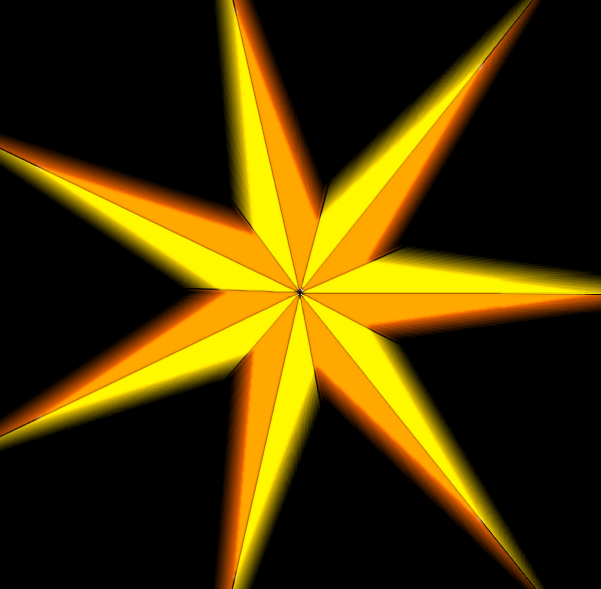This is the codelab:

```void Render(Surface dst, Surface src, Rectangle rect)
{
int n =7;
float a =0;
float b =0;
int R1 =290;
int R2=R1/4;
int ray =3;

PdnRegion selectionRegion = EnvironmentParameters.GetSelection(src.Bounds);
Rectangle selection = this.EnvironmentParameters.GetSelection(src.Bounds).GetBoundsInt();

long CenterX = (long)(((selection.Right - selection.Left) / 2) + selection.Left);
long CenterY = (long)(((selection.Bottom - selection.Top) / 2) + selection.Top);
int pt = 0;

float r = (float)(Math.Min(CenterX - selection.Left, CenterY - selection.Top));

// Half Width is integer... So workWidth is even
long workHWidth = (int)r + 1;
long workWidth = (workHWidth + 1) * 2;

long workPoints = workWidth * workWidth;

bool[] MapDraw = new bool[workPoints];
for (int j = 0; j < workPoints; ++j) { MapDraw[j] = false; }

ColorBgra PrimColor = (ColorBgra)EnvironmentParameters.PrimaryColor;

float x1, y1, x2, y2;
long x0 = CenterX;
long y0 = CenterY;

float vx1, vy1, vx2, vy2;
float aa  = (float)(Math.PI / n);
float sina = (float)Math.Sin(aa);
float cosa  = (float)Math.Cos(aa);
float sin2a  = (float)(2 * cosa * sina);
float cos2a  = 1 - 2 * sina * sina;
aa = (float)(Math.PI * a / 180);
float sinb  = (float)Math.Sin(aa);
float cosb = (float)Math.Cos(aa);
aa = (float)(Math.PI * b / 180);
float sinc  = (float)Math.Sin(aa);
float cosc  = (float)Math.Cos(aa);
vx1 = R1 * cosb;
vy1 = R1 * sinb;
vx2 = (R2 * cosa);
vy2 = (R2 * sina);
float vtx, vty;
vtx = vx2;
vty = vy2;
vtx = (vx2 * cosc + vy2 * sinc);
vty = (-vy2 * sinc + vy2 * cosc);
vx2 = vtx;
vy2 = vty;
vtx = (vx1 * cosc + vy1 * sinc);
vty = (-vx1 * sinc + vy1 * cosc);
vx1 = vtx;
vy1 = vty;
x1 = (float)(x0 + vx1);
y1 = (float)(y0 + vy1);
x2 = (float)(x0 + vx2);
y2 = (float)(y0 + vy2);

// ================== FIRST LOOP ==========================
// Fill binary table with the plotted polygone
for (int i = 1; i < n+1; i++)
{
if (ray != 2)
{
DrawLine(x0, y0, x1, y1, MapDraw, workWidth, true);
}
if (ray != 1)
{
DrawLine(x1, y1, x2, y2, MapDraw, workWidth, true);
}

vtx = vx2;
vty = vy2;
vx2 = (vtx * cos2a + vty * sin2a);
vy2 = (-vtx * sin2a + vty * cos2a);
x2 = x0 + vx2;
y2 = y0 + vy2;
if (ray != 1)
{
DrawLine(x2, y2, x1, y1, MapDraw, workWidth, true);
}
vtx = vx1;
vty = vy1;
vx1 = (vtx * cos2a + vty * sin2a);
vy1 = (-vtx * sin2a + vty * cos2a);
x1 = x0 + vx1;
y1 = y0 + vy1;
}

// ================== SECOND LOOP ==========================
// Final rendering
for (int y = rect.Top; y < rect.Bottom; y++)
{
for (int x = rect.Left; x < rect.Right; x++)
{
if (selectionRegion.IsVisible(x, y))
{
dst[x, y] = src[x, y];
if (Math.Abs(y - CenterY) <= (int)(workHWidth))
{
if (Math.Abs(x - CenterX) <= (int)(workHWidth))
{
pt = (int)((x - CenterX + (workHWidth)) + workWidth * (y - CenterY + (workHWidth)));
if (MapDraw[pt] == true)
{
dst[x, y] = PrimColor;
}
}
}
}
}
}
}
private void DrawLine(float x1, float y1, float x2, float y2, Boolean[] MapDraw, float workWidth, bool lines)
{
int roundx, roundy, i, pt;
float xi, yi, dx, dy, x, y, l;
float arrondi = 0.0f;
if (lines)
{
dx = x2 - x1;
dy = y2 - y1;

if (Math.Abs(dx) > Math.Abs(dy))
{
l = dx;
}
else
{
l = dy;
}

if (l < 0)
{
DrawLine(x2, y2, x1, y1, MapDraw, workWidth, lines);
}
else
{
xi = dx / l;
yi = dy / l;

x = x1;
y = y1;

roundx = (int)(x + arrondi);
roundy = (int)(y + arrondi);
pt = (int)(roundx + workWidth * roundy);
if (pt < 0) pt = 1;
if (pt > (workWidth * workWidth)) pt = 1;
MapDraw[pt] = true;

for (i = 0; i <= l; i++)
{
x = x + xi;
y = y + yi;

roundx = (int)(x + arrondi);
roundy = (int)(y + arrondi);
pt = (int)(roundx + workWidth * roundy);
if (pt < 0) pt = 1;
if (pt > (workWidth * workWidth)) pt = 1;
MapDraw[pt] = true;
}
}
}
else
{
roundx = (int)(x2 + arrondi);
roundy = (int)(y2 + arrondi);
pt = (int)(roundx + workWidth * roundy);
if (pt < 0) pt = 1;
if (pt > (workWidth * workWidth)) pt = 1;
MapDraw[pt] = true;
}
}
```My DeviantArt | My Pictorium | My Plugins | Donate via Paypal

##### Share on other sites

Wow! You makes it looks very pro.

I am coming up with a new idea, a plugin to make gears -- in fact, it should be generally to be a multiple folded center point symmetric, with the target curve represents by bezier curve (therefore we are dealing with points in fact and finally connect them by bezier curve) --- need some restriction to prevent it looks stupid.

BTW, I am sorry the vector operation is not flawless -- even it is fast to calculate a 3000 vertex star, the end can't be closed because of the round off error. Only cos and sin can survive.

```        Dim n As Integer = 7
Dim a As Double = 30.0
Dim b As Double = 0.0
Dim R1 As Integer = 250 'CInt(Math.Min(Me.ClientSize.Width, Me.ClientSize.Height) / 2 - 4)
Dim R2 As Integer = 200 'CInt(R1 / 2)
Dim ray As Integer = 2

Dim x1, y1, x2, y2, xt, yt As Integer
Dim x0 As Integer = 300 ' CInt(Me.ClientSize.Width / 2)
Dim y0 As Integer = 300 ' CInt(Me.ClientSize.Height / 2)
Dim aa As Single = a * Math.PI / 180
Dim bb As Single = b * Math.PI / 180
Dim cc As Single = Math.PI / n
xt = x0 + R2 * Math.Cos(bb - cc)
yt = x0 + R2 * Math.Sin(bb - cc)

Dim i As Integer
For i = 0 To n Step 1
x1 = x0 + R1 * Math.Cos(aa + bb + 2 * i * cc)
y1 = x0 + R1 * Math.Sin(aa + bb + 2 * i * cc)
x2 = x0 + R2 * Math.Cos(bb + (2 * i + 1) * cc)
y2 = x0 + R2 * Math.Sin(bb + (2 * i + 1) * cc)

If ray = 2 Or ray = 3 Then
gr.DrawLine(Pens.Red, x1, y1, x2, y2)
gr.DrawLine(Pens.Red, x1, y1, xt, yt)
End If

If ray = 1 Or ray = 3 Then
gr.DrawLine(Pens.Red, x0, y0, x1, y1)
gr.DrawLine(Pens.Red, x0, y0, x2, y2)
End If
xt = x2
yt = y2
Next
```

The bezier curve to draw gear ends with result, might be used somewhere else```   Private Sub form2_paint(ByVal sender As Object, ByVal e As PaintEventArgs) Handles MyBase.Paint
Dim gr As Graphics = e.Graphics
Dim n As Integer = 12
Dim i As Integer
Dim R1, R2 As Integer
Dim x0 As Integer = 150, y0 As Integer = 150
R1 = 100
R2 = 70
Dim s As Integer
s = 1

' local variables
Dim A1, A2, A3, A4 As Double
Dim x1, y1, x2, y2, x3, y3, x4, y4, R As Integer
A1 = Math.PI / n
A2 = Math.PI * 0 / 180
x1 = x0 + R1 * Math.Cos(A2)
y1 = y0 + R1 * Math.Sin(A2)
A3 = A2 + s * Math.PI / 3
x2 = x0 + 2 * R1 * Math.Cos(A3)
y2 = y0 + 2 * R1 * Math.Sin(A3)
R = R1

For i = 1 To 2 * n
If R = R1 Then
R = R2
Else
R = R1
End If
A3 = i * A1 + A2
x3 = x0 + R * Math.Cos(A3)
y3 = y0 + R * Math.Sin(A3)
A4 = A3 - s * Math.PI / 3
x4 = x0 + 2 * R * Math.Cos(A4)
y4 = y0 + 2 * R * Math.Sin(A4)
gr.DrawBezier(Pens.Black, x1, y1, x2, y2, x4, y4, x3, y3)
x1 = x3
y1 = y3
x2 = 2 * x3 - x4
y2 = 2 * y3 - y4
'gr.DrawLine(Pens.Black, x0, y0, x1, y1)
'gr.DrawLine(Pens.Black, x1, y1, x2, y2)

Next

gr.Dispose()

End Sub
```

##### Share on other sites
• 2 weeks later...

I've created another plugin based on Ghost_ARCHER visual basic code (the first version, not the one with bezier curves).(picture from Ghost_ARCHER)

It's allow you to draw stars (different than Boltbait polygon http://paintdotnet.12.forumer.com/viewtopic.php?t=2580 )

Anti-Aliasing methode (checkbox).

Use the actual brush width.

here is the DLL

____________________________________________

To install:

Close Paint.net

Unzip and (re)place the DLL in your Effect folder usualy: C:\Program Files\Paint.NET\Effects

____________________________________________

here is the Sources

An example where I've used this plugin:

Another desktop abstract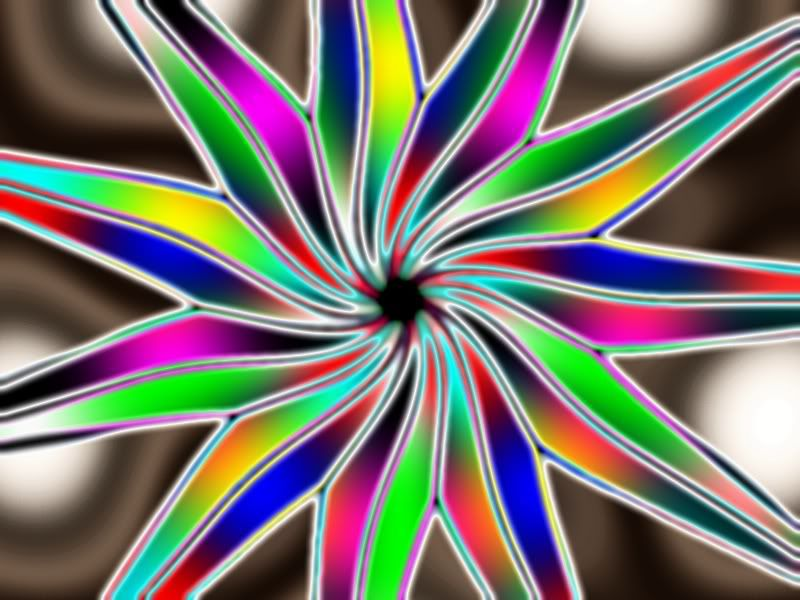My DeviantArt | My Pictorium | My Plugins | Donate via Paypal

##### Share on other sitesLook at the first post of this topic for the lastest version of the SpiroShape plugin.

http://paintdotnet.12.forumer.com/viewtopic.php?p=16888#16888My DeviantArt | My Pictorium | My Plugins | Donate via Paypal

##### Share on other sitesCool plugin man!

Take responsibility for your own intelligence.-Rick Brewster

##### Share on other sites

Thanks...

No plan to change it.

I'm working on another one with bezier's curves...My DeviantArt | My Pictorium | My Plugins | Donate via Paypal

##### Share on other sites
• 3 weeks later...

geez... you guys speak a whole other language...

##### Share on other sites

Hey guys...came up with this odd result after playing with the effects here:I created a flower-shape (ala http://paintdotnet.12.forumer.com/viewtopic.php?t=3731), selected the center, deleted it, and then tried to add a star in the middle...it turned out that it also added some sort of strange black border as well. Is this a glitch perhaps?## Join the conversation

You can post now and register later. If you have an account, sign in now to post with your account.×   Pasted as rich text.   Paste as plain text instead

Only 75 emoji are allowed.

×   Your previous content has been restored.   Clear editor

×   You cannot paste images directly. Upload or insert images from URL.

×
×